# Stability in the presence of persistently acting perturbations

A property of the solution,, of an initial value problem(*)

which consists of the following. For everythere is a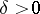such that for every point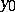satisfying the inequality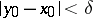, and every mapping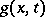satisfying the conditions

a)and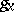are continuous on the set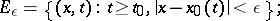b)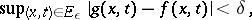the solutionof the initial value problemis defined for alland satisfies the inequality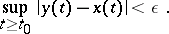Bohl's theorem . Suppose that the initial value problem (*) has a solution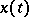,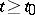, satisfying the conditions:)and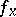are continuous onfor some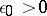;);) the mappingis differentiable with respect toat the pointfor, uniformly with respect to, i.e.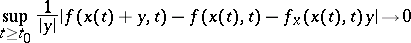Then in order that this solution of the initial value problem be stable in the presence of persistently acting perturbations, it is necessary and sufficient that the upper singular exponent (cf. Singular exponents) of the system of variational equations of the system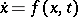along the solutionbe less than zero.

If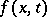does not depend on(an autonomous system) and the solutionis periodic or constant, and also ifis periodic inand the solutionis periodic with the same (or a commensurable) period or is a constant, then: 1) the condition stated in Bohl's theorem on uniform differentiability is superfluous (it follows from the remaining conditions of the theorem); and 2) the upper singular exponent of the system of variational equations of the systemalong the solutioncan be calculated efficiently.

How to Cite This Entry:
Stability in the presence of persistently acting perturbations. V.M. Millionshchikov (originator), Encyclopedia of Mathematics. URL: http://encyclopediaofmath.org/index.php?title=Stability_in_the_presence_of_persistently_acting_perturbations&oldid=17291
This text originally appeared in Encyclopedia of Mathematics - ISBN 1402006098